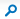# MathsShare

 This Site: MathsShareMathsShare > Puzzles > Water and Wine > Water and wine

## Puzzles: Water and wine### Body

Since after the two transfers there is still a equal volume in each glass, an equal amount of water must now be in the wine as there is wine in the water!

Mathematically:
Let V be the volume of water in the first glass. V is also the volume of wine in the second glass. Let S be the volume of one spoonful.

After the first transfer the volumes are:

In the 1st glass: Water = V-S, Wine=0
In the 2nd glass: Water = S, Wine=V
The fraction of water in the second glass is S/(S+V)

Therefore the second transfer consists of: Water = SxS/(S+V), and Wine= S - SxS/(S+V)

And so after the second transfer:
In the 1st glass: Water = V - S + SxS/(S+V), Wine = S - SxS/(S+V)
In the 2nd glass: Water = S - SxS/(S+V), Wine = V - S + SxS/(S+V)

There is the same amount of wine in the water as there is water in the wine.

Try it with V=90 and S=10:

After first transfer, first glass is (80 water, 0 wine), second glass is (10 water, 90 wine) which is 10% water. So the second transfer is of (1 water, 9 wine) giving a final position: First glass (81 water, 9 wine), second glass (9 water, 81 wine).

### AttachmentsContent Type: Message
 Created at 20/05/2013 04:55 PM by John Rowland Last modified at 20/05/2013 04:57 PM by John Rowland